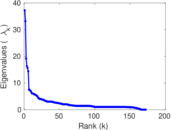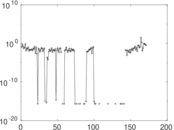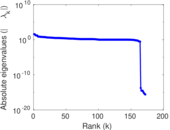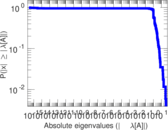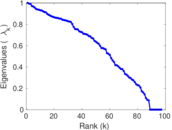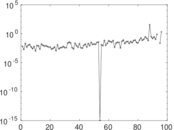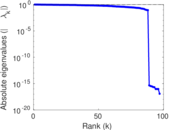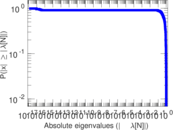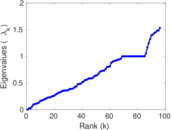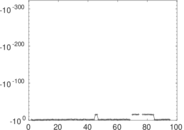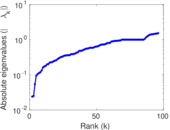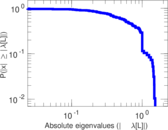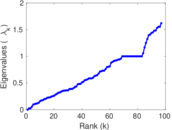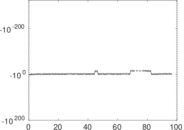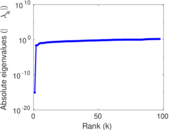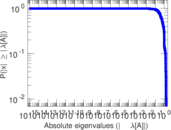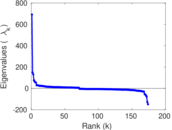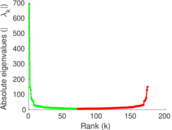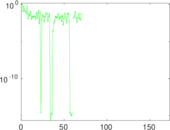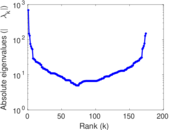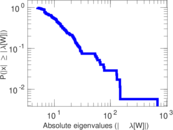# Wikiquote edits (uz)

This is the bipartite edit network of the Uzbek Wikiquote. It contains users and pages from the Uzbek Wikiquote, connected by edit events. Each edge represents an edit. The dataset includes the timestamp of each edit.

 Code `quz` Internal name `edit-uzwikiquote` Name Wikiquote edits (uz) Data source http://dumps.wikimedia.org/ AvailabilityDataset is available for download Consistency checkDataset passed all tests Category Authorship network Dataset timestamp 2017-10-20 Node meaning User, article Edge meaning Edit Network formatBipartite, undirected Edge typeUnweighted, multiple edges Temporal dataEdges are annotated with timestamps

## Statistics

 Size n = 704 Left size n1 = 174 Right size n2 = 530 Volume m = 1,221 Unique edge count m̿ = 800 Wedge count s = 21,778 Claw count z = 1,095,395 Cross count x = 48,695,682 Square count q = 2,099 4-Tour count T4 = 105,644 Maximum degree dmax = 202 Maximum left degree d1max = 202 Maximum right degree d2max = 37 Average degree d = 3.468 75 Average left degree d1 = 7.017 24 Average right degree d2 = 2.303 77 Fill p = 0.008 674 91 Average edge multiplicity m̃ = 1.526 25 Size of LCC N = 489 Diameter δ = 13 50-Percentile effective diameter δ0.5 = 3.984 10 90-Percentile effective diameter δ0.9 = 7.200 57 Median distance δM = 4 Mean distance δm = 4.777 76 Gini coefficient G = 0.662 516 Balanced inequality ratio P = 0.235 463 Left balanced inequality ratio P1 = 0.216 216 Right balanced inequality ratio P2 = 0.298 116 Relative edge distribution entropy Her = 0.865 730 Power law exponent γ = 3.899 84 Tail power law exponent γt = 2.361 00 Tail power law exponent with p γ3 = 2.361 00 p-value p = 0.011 000 0 Left tail power law exponent with p γ3,1 = 2.171 00 Left p-value p1 = 0.363 000 Right tail power law exponent with p γ3,2 = 2.771 00 Right p-value p2 = 0.005 000 00 Degree assortativity ρ = −0.300 901 Degree assortativity p-value pρ = 3.322 59 × 10−18 Spectral norm α = 37.229 0 Algebraic connectivity a = 0.023 789 5 Spectral separation |λ1[A] / λ2[A]| = 1.121 59 Controllability C = 371 Relative controllability Cr = 0.530 758

## Plots

### Fruchterman–Reingold graph drawing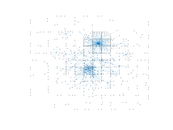### Degree distribution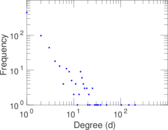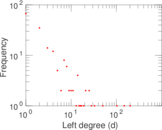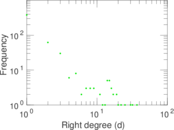### Cumulative degree distribution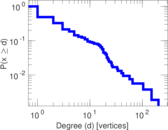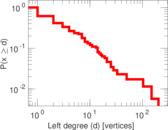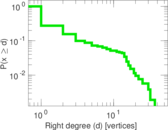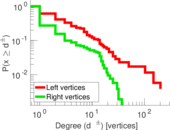### Lorenz curve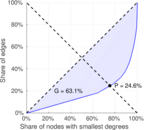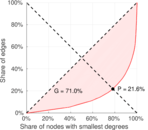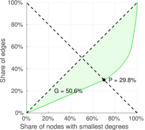### Spectral distribution of the adjacency matrix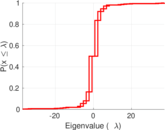### Spectral distribution of the normalized adjacency matrix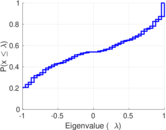### Spectral distribution of the Laplacian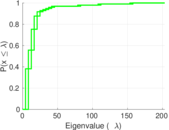### Spectral graph drawing based on the adjacency matrix### Spectral graph drawing based on the Laplacian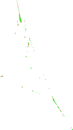### Spectral graph drawing based on the normalized adjacency matrix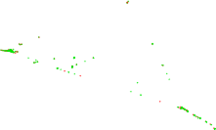### Degree assortativity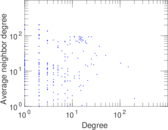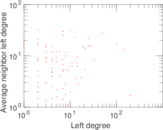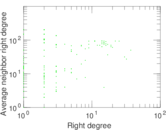### Zipf plot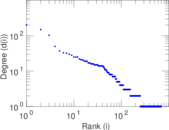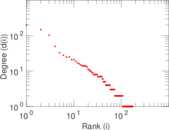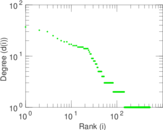### Hop distribution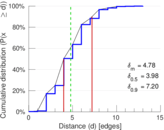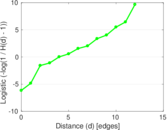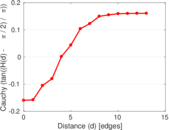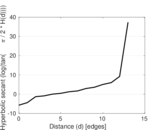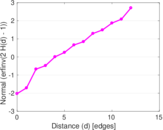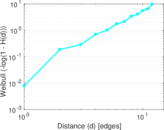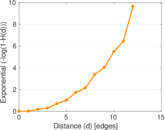### Double Laplacian graph drawing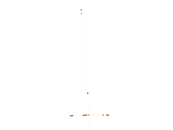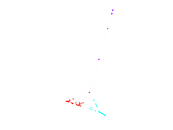### Delaunay graph drawing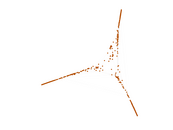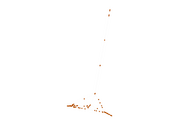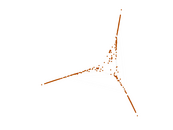### Edge weight/multiplicity distribution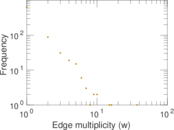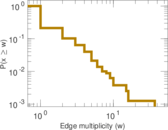### Temporal distribution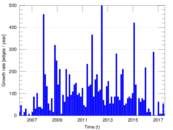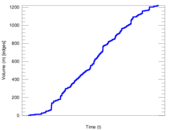### Temporal hop distribution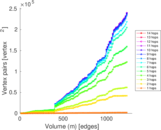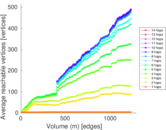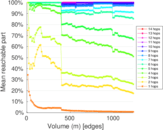### Diameter/density evolution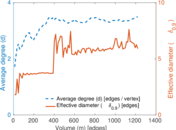### Matrix decompositions plots